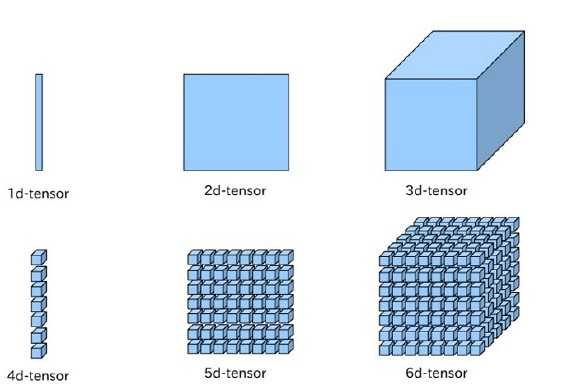#### 向量 vector

$x_1$是向量$\bf x$的第一个元素。当需要明确向量中的每一个元素时，可以使用方括号包围的一个纵列来表示，如：$\bf x \rm = \begin{bmatrix} x_1 \ x_2 \\vdots \x_n \end{bmatrix}$。有的时候需要明确的索引一个向量中的部分元素，可以先定义一个索引集合，再用索引集合做向量的下表，来表达。如：$s={1,3,6}$，然后写作$\bf x_s$。可以通过补集的形式明确限定一组元素，如除了$x_1$之外的所有元素，表示为$\bf x_{-1}$。除了$x_1,x_3,x_6$之外的一个向量可以表示为$\bf x{-_s}$。

#### 张量 tensor

• 张量是一个这样的量，它能在不同的参考系中按照特定的法则进行变换。它的物理意义是使的物理公式的表达独立于参考系[^1]。

比如两个粒子1和2经过散射变成了3和4。

在a参考系中，能动量守恒是$(E_{a,1},P_{a,1})+(E_{a,2},P_{a,2})=(E_{a,3},P_{a,3})+(E_{a,4},P_{a,4})$

在b参考系中，能动量守恒是$(E_{b,1},P_{b,1})+(E_{b,2},P_{b,2})=(E_{b,3},P_{b,3})+(E_{b,4},P_{b,4})$

那么可以用张量表达为$T_1+T_2=T_3+T_4$

• 张量是多重线性函数，$T(v_1,v_2,\dots,v_r)$，输入r个向量，输出一个数，r称为张量的阶数。多重线性是指张量对于每个参数都是线性的[^2]。线性的本质是齐次性和可加性，也可以简单理解为张量与每个参数的关系函数，画成图像的话是一条直线。

• 在机器学习领域，张量可以更简化的理解为N维数组。0阶张量就是标量，1阶张量就是向量，2阶张量就是矩阵，3阶及以上就没有特殊的称呼，直接称为N阶张量。下图来源于csdn[^3]。#### END

[^1]: 知乎-andrew shen
[^2]: 知乎-郭诚
[^3]: 高维数组的机器学习